Mathematical Models in Engineering

Published: 31 March 2020

# Dynamics of a single mass vibrating system impacting into a deformable support

K. Ragulskis1
L. Ragulskis2
1Kaunas University of Technology, K. Donelaičio Str. 73, LT-44249, Kaunas, Lithuania
2Vytautas Magnus University, Vileikos Str. 8, LT-44404, Kaunas, Lithuania
Corresponding Author:
K. Ragulskis
Views 32

#### Abstract

The investigated system comprises a mass attached by a deformable link to a fixed foundation, and an elastic-dissipative limiter of motion of that mass. Such types of systems are widely used in different technological devices and machines. This paper is devoted for the improvement of dynamical qualities of such systems. Free and forced stationary harmonic vibrations as well as the qualitative parameters of motions of the system are analyzed in this paper. Characteristics of vibrations are determined using analytical and numerical techniques. It is determined that for the case of zero fastening the values of eigenfrequencies of the system do not depend on the amplitude of excitation. Then the system has an infinite number of multiple eigenfrequencies. In the case of forced harmonic excitation single valued stable motions exist in the vicinity of the resonance. This gives rise to some qualities of the system which are useful in practical applications.

## 1. Introduction

This paper is focused on a nonlinear system which is characterized by two following features: first – the values of eigenfrequencies do not depent on the amplitude of excitation, and the second – an infinite number of eigenfrequencies does exist. The dynamics of such kind of systems has not been investigated in the existing literature.

Essentially nonlinear systems, two-dimensional transmissions, their dynamics and vibrations are analysed in . Dynamics of vibromotors for precision microrobots is described in . Resonances in nonlinear vibrating systems are investigated in . Impact dynamics under periodic and transient excitations are analysed in . Stabilization of periodic nonlinear systems is investigated in . Analysis of behaviour of mechanical systems with impacts is performed in . Periodic orbits of mechanical systems with impacts are analysed in . Behaviour of a vibro-impact nonlinear energy sink is investigated in . The dynamics of a particle impact with a wall is analysed in .

The investigated system is shown in Fig. 1, where $m$, $C$ and $H$ are the mass of the main vibrating part, the coefficients of stiffness and of viscous friction respectively; while ${C}_{0}$ and ${H}_{0}$ are the coefficients of stiffness and viscous friction of the limiter. The positions $OO$ and ${O}_{0}{O}_{0}$ are positions of static equilibrium of the contact surfaces of the main part $x$ and of the limiter ${x}_{0}$ respectively. By ${x}_{s}$ the fastening of the system in the statical position is denoted. Positive values of $x$ and ${x}_{0}$ are counted to the right from the position ${O}_{0}{O}_{0}$.

The investigated system is described by the following differential equations:

1
2
3

where:

4

Known methods are used for analytical and numerical investigations.

Fig. 1Schematic representation of the system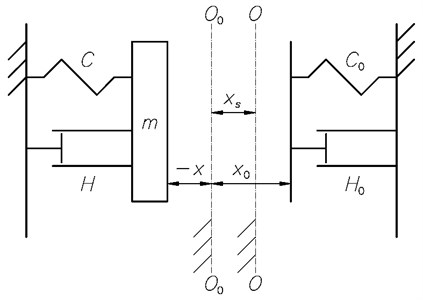## 2. Free damped vibrations, that is when ${\mathbit{x}}_{\mathbit{s}}=\mathbit{f}=0$

Case $x={x}_{0},$ that is according to Eq. (1) it is assumed that this takes place in the interval:

5
$t\in \left[0,{T}_{a}\right),$

and at $t=0:x=0$, $\stackrel{˙}{x}={\stackrel{˙}{x}}^{-}$, and at $t={T}_{a}$ goes from the position $x={x}_{0}$ to the position $x<{x}_{0}$ and:

6
$\stackrel{¨}{x}+2\left(h+{h}_{0}\right)\stackrel{˙}{x}+\left({p}^{2}+{p}_{0}^{2}\right)x=0,$

has the solution:

7
$x={C}_{1}{e}^{{\lambda }_{1}t}+{C}_{2}{e}^{{\lambda }_{2}t},$

where:

8
${\lambda }^{2}+2\left(h+{h}_{0}\right)\lambda +\left({p}^{2}+{p}_{0}^{2}\right)=0,$

where it is denoted:

9

${C}_{1}$ and ${C}_{2}$ are found from the initial conditions of motion Eq. (7) at $t=0:$

10
$0={e}^{-\left(h+{h}_{0}\right)\cdot 0}{C}_{1}\mathrm{c}\mathrm{o}\mathrm{s}\sqrt{0}⇒{C}_{1}=0,$
11

That is:

12
13
14

At $t={T}_{a}$ the Eq. (3) is not valid and Eqs. (2) and (5) are valid: into Eq. (2) solutions Eqs. (12) and (13) are substituted by assuming $t={T}_{a}$, that is the moment of disconnection of $x$ and ${x}_{0}:$

15

The same ${T}_{a}$ must be obtained by substituting the solutions $\stackrel{˙}{x}$ and $x$ at ${T}_{a}$ into Eq. (1), that is:

Further at $t\in \left({T}_{a},{T}_{k}\right)$ motion takes place according to the Eqs. (2) and (3) with initial parameters:

16

The solution of Eq. (2) in the interval:

17
$t\in \left({T}_{a},{T}_{0}\right),$

where:

18

is:

19
$x={e}^{-h\left(t-{T}_{a}\right)}\left[{D}_{1}\mathrm{c}\mathrm{o}\mathrm{s}\sqrt{{p}^{2}-{h}^{2}}\left(t-{T}_{a}\right)+{D}_{2}\mathrm{s}\mathrm{i}\mathrm{n}\sqrt{{p}^{2}-{h}^{2}}\left(t-{T}_{a}\right)\right],$

where ${D}_{1}$, ${D}_{2}$ are found according to the initial conditions Eq. (14):

20
$x\left({T}_{a}\right)={D}_{1},$
$\stackrel{˙}{x}\left({T}_{a}\right)=-h{D}_{1}\mathrm{c}\mathrm{o}\mathrm{s}\sqrt{{p}^{2}-{h}^{2}}0-h\sqrt{{p}^{2}-{h}^{2}}{D}_{2}\mathrm{s}\mathrm{i}\mathrm{n}\sqrt{{p}^{2}-{h}^{2}}0$

${D}_{1}=x\left({T}_{0}\right),$
$\stackrel{˙}{x}\left({T}_{a}\right)=-hx\left({T}_{a}\right)-\sqrt{{p}^{2}-{h}^{2}}{D}_{2}⇒{D}_{2}=-\frac{\stackrel{˙}{x}\left({T}_{a}\right)}{\sqrt{{p}^{2}-{h}^{2}}}-\frac{h}{\sqrt{{p}^{2}-{h}^{2}}}x\left({T}_{a}\right).$

Eq. (20) minus Eq. (19) gives:

21
$x={e}^{-h\left(t-{T}_{a}\right)}\left\{x\left({T}_{a}\right)\mathrm{c}\mathrm{o}\mathrm{s}\sqrt{{p}^{2}-{h}^{2}}\left(t-{T}_{a}\right)\right\$

$\stackrel{˙}{x}={e}^{-h\left(t-{T}_{a}\right)}\left\{-hx\left({T}_{a}\right)\mathrm{c}\mathrm{o}\mathrm{s}\sqrt{{p}^{2}-{h}^{2}}\left(t-{T}_{a}\right)\right\$

$\stackrel{˙}{x}={e}^{-h\left(t-{T}_{a}\right)}\left\{-\left[2hx\left({T}_{a}\right)+\stackrel{˙}{x}\left({T}_{a}\right)\right]\mathrm{c}\mathrm{o}\mathrm{s}\sqrt{{p}^{2}-{h}^{2}}\left(t-{T}_{a}\right)\right\$

It is assumed that when $t=T:$

22

In the Eqs. (20), (21) by assuming $t=T,$ that is $t-{T}_{a}={T}_{b}:$

23
$0={e}^{-h\left(T-{T}_{a}\right)}\left\{x\left({T}_{a}\right)\mathrm{c}\mathrm{o}\mathrm{s}\sqrt{{p}^{2}-{h}^{2}}\left(T-{T}_{a}\right)\right\$
24
$\stackrel{˙}{x}={e}^{-h\left(T-{T}_{a}\right)}\left\{-\left[2hx\left({T}_{a}\right)+\stackrel{˙}{x}\left({T}_{a}\right)\right]\mathrm{c}\mathrm{o}\mathrm{s}\sqrt{{p}^{2}-{h}^{2}}\left(T-{T}_{a}\right)\right\$

From Eq. (23) $T$ is determined and from Eq. (24) ${\stackrel{˙}{x}}^{-}\left(T\right).$

The coefficient of restitution of velocity of the support is:

25
${R}_{h}=\frac{\stackrel{˙}{x}\left({T}_{a}\right)}{{x}^{-}},$

by taking into account Eqs. (13) and (15):

26

or:

27

where ${T}_{0}$ is determined by the Eq. (15).

## 3. Vibrations of the conservative system

In this case in the Eqs. (1-3) it is assumed that:

28

Two intervals of time are investigated: the first one:

29

and the second one:

30

In case of the first interval it is assumed:

31
32

According to the Eqs. (1), (28), (29), (30) it is obtained:

33
$x=-{D}_{1}\mathrm{c}\mathrm{o}\mathrm{s}\sqrt{{p}^{2}+{p}_{0}^{2}}t+{D}_{2}\mathrm{s}\mathrm{i}\mathrm{n}\sqrt{{p}^{2}+{p}_{0}^{2}}t+{D}_{1},$
34
$\frac{\stackrel{˙}{x}}{\sqrt{{p}^{2}+{p}_{0}^{2}}}={D}_{1}\mathrm{s}\mathrm{i}\mathrm{n}\sqrt{{p}^{2}+{p}_{0}^{2}}t+{D}_{2}\mathrm{c}\mathrm{o}\mathrm{s}\sqrt{{p}^{2}+{p}_{0}^{2}}t,$

where:

35

At $t={\stackrel{-}{T}}_{1}$, by taking into account Eqs. (32-35), it is obtained:

36
$\mathrm{t}\mathrm{a}\mathrm{n}\sqrt{{p}^{2}+{p}_{0}^{2}}{\stackrel{-}{T}}_{1}=\frac{2{X}_{s}}{1-{X}_{s}^{2}},$

where:

37
${X}_{s}=\frac{1}{\sqrt{1+{\left(\frac{{p}_{0}}{p}\right)}^{2}}}\cdot \frac{p{x}_{s}}{{\stackrel{˙}{x}}^{-}}.$

In case of the second interval by taking into account Eq. (30) it is assumed:

38
39

According to the Eqs. (2), (28), (38), (39) it is obtained:

40
$x=-{x}_{s}\mathrm{c}\mathrm{o}\mathrm{s}p\left(t-{\stackrel{-}{T}}_{1}\right)-\frac{{\stackrel{˙}{x}}^{-}}{p}\mathrm{s}\mathrm{i}\mathrm{n}\left(t-{\stackrel{-}{T}}_{1}\right)+{x}_{s},$
41
$\frac{\stackrel{˙}{x}}{\stackrel{-}{p}}={x}_{s}\mathrm{s}\mathrm{i}\mathrm{n}p\left(t-{T}_{1}\right)-\frac{{\stackrel{˙}{x}}^{-}}{p}\mathrm{c}\mathrm{o}\mathrm{s}p\left(t-{\stackrel{-}{T}}_{1}\right).$

At $t={\stackrel{-}{T}}_{1}+{\stackrel{-}{T}}_{2},$ by taking into account Eqs. (39-41), it is obtained:

42
$\mathrm{t}\mathrm{a}\mathrm{n}p{\stackrel{-}{T}}_{2}=2\frac{\frac{p{x}_{s}}{\omega }}{\left(\frac{p{x}_{s}}{{\stackrel{˙}{x}}^{-}}\right)-1}.$

Period of the system:

43
$\stackrel{-}{T}={\stackrel{-}{T}}_{1}+{\stackrel{-}{T}}_{2},$

where ${\stackrel{-}{T}}_{1}$ and ${\stackrel{-}{T}}_{2}$ are determined according to the Eqs. (36), (37), (42).

In separate case, when:

44
${x}_{s}=0,$

according to the Eqs. (36), (37), (42) it is obtained:

45

that is:

46
$T=\left[1+\frac{1}{\sqrt{1+{\left(\frac{{p}_{0}}{p}\right)}^{2}}}\right]\frac{\pi }{p}.$

## 4. Numerical investigation of the damped system

It is assumed that $x\left(0\right)=\text{0}$, $\stackrel{˙}{x}\left(0\right)=\text{1}$, ${x}_{0}\left(0\right)=\text{0}$, $c=\text{1}$, ${c}_{0}=\text{1}.$ Results for $h={h}_{0}=$ 0.2 are represented by a thin line; for $h={h}_{0}=$ 0.1 are represented by a line of medium thickness; for $h={h}_{0}=$ 0.001 are represented by a thick line. The force is calculated as $P={h}_{0}{\stackrel{˙}{x}}_{0}+{c}_{0}{x}_{0}.$

Results of computations at ${x}_{s}=$ –0.2 are depicted in Fig. 2.

Fig. 2Dynamics of the system at xs= –0.2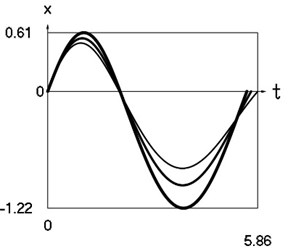a) Displacement as function of time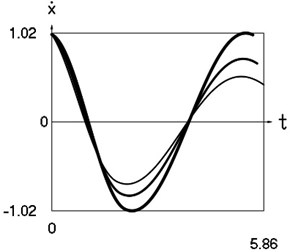b) Velocity as function of time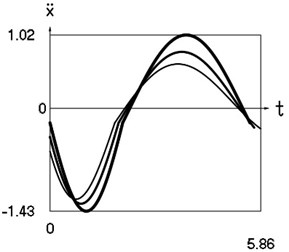c) Acceleration as function of time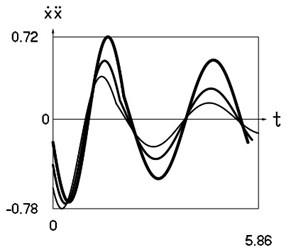d) Velocity multiplied by acceleration as function of time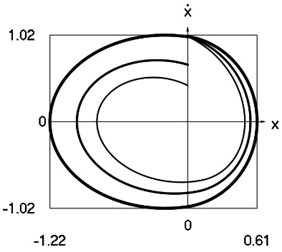e) Phase trajectory: velocity as function of displacement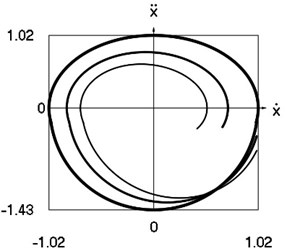f) Phase trajectory: acceleration as function of velocity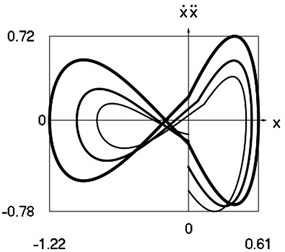g) Phase trajectory: velocity multiplied by acceleration as function of displacement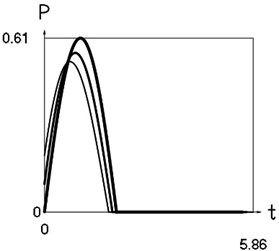h) Force as function of time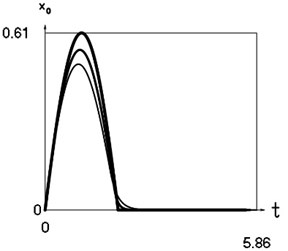i) Displacement of support as function of time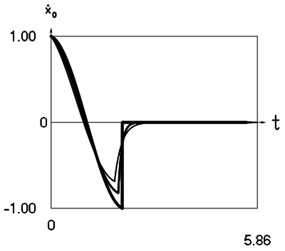j) Velocity of support as function of time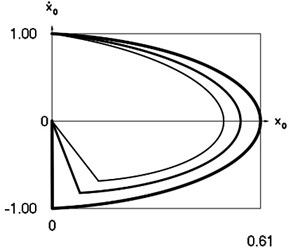k) Phase trajectory: velocity of support as function of displacement of support

Analogously, results of computations at ${x}_{s}=0$ are depicted in Fig. 3.

Fig. 3Dynamics of the system at xs=0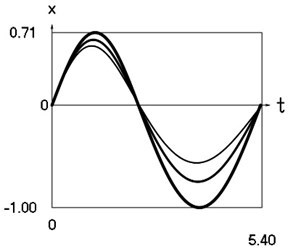a) Displacement as function of time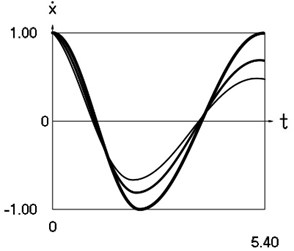b) Velocity as function of time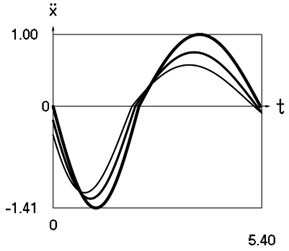c) Acceleration as function of time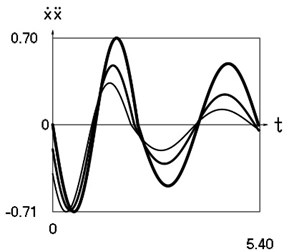d) Velocity multiplied by acceleration as function of time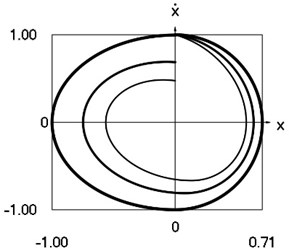e) Phase trajectory: velocity as function of displacement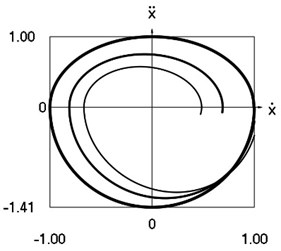f) Phase trajectory: acceleration as function of velocity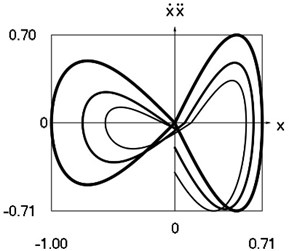g) Phase trajectory: velocity multiplied by acceleration as function of displacement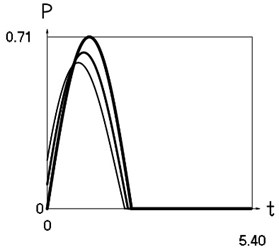h) Force as function of time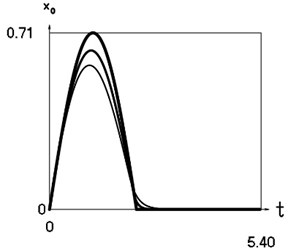i) Displacement of support as function of time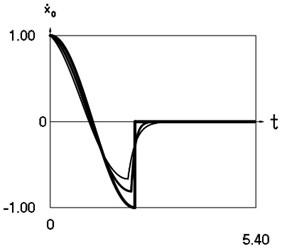j) Velocity of support as function of time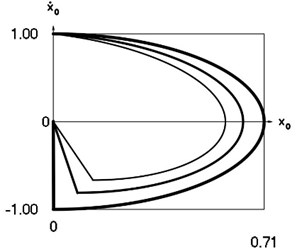k) Phase trajectory: velocity of support as function of displacement of support

Finally, results of computations at ${x}_{s}=$ 0.2 are depicted in Fig. 4.

Fig. 4Dynamics of the system at xs= 0.2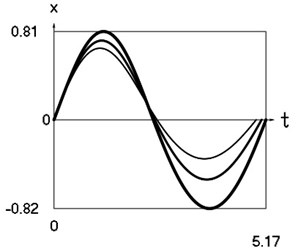a) Displacement as function of time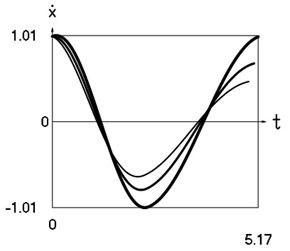b) Velocity as function of time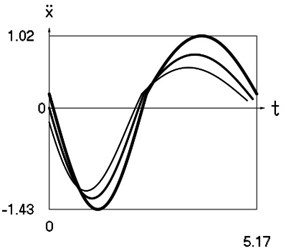c) Acceleration as function of time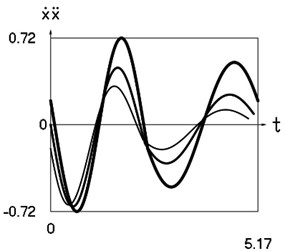d) Velocity multiplied by acceleration as function of time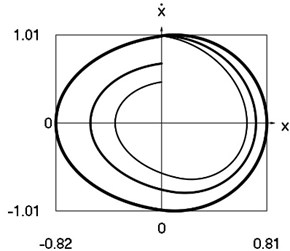e) Phase trajectory: velocity as function of displacement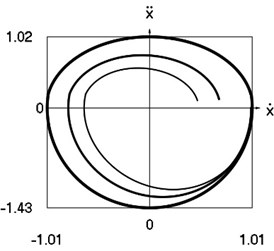f) Phase trajectory: acceleration as function of velocity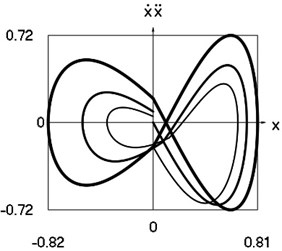g) Phase trajectory: velocity multiplied by acceleration as function of displacement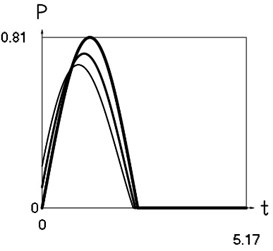h) Force as function of time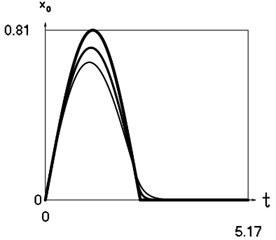i) Displacement of support as function of time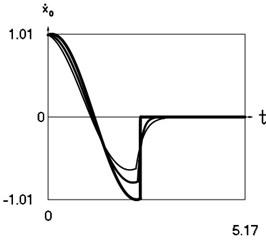j) Velocity of support as function of time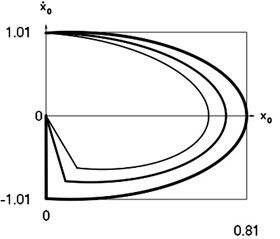k) Phase trajectory: velocity of support as function of displacement of support

## 5. Numerical investigation of the conservative system

It is assumed that $x\left(0\right)=$ 0, $\stackrel{˙}{x}\left(0\right)=$ 1, $c=$ 1, ${c}_{0}=$ 1. Results for ${x}_{s}=$ –1 are represented by a thin line; for ${x}_{s}=0$ are represented by a line of medium thickness; for ${x}_{s}=$ 1 are represented by a thick line. The force is calculated as $P={c}_{0}{x}_{0}.$

Fig. 5The dynamics of the conservative system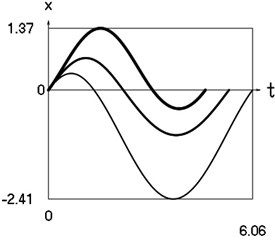a) Displacement as function of time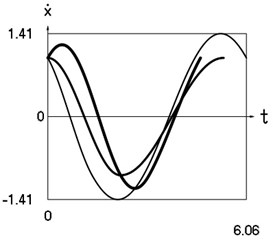b) Velocity as function of time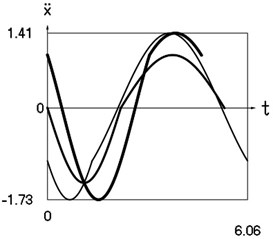c) Acceleration as function of time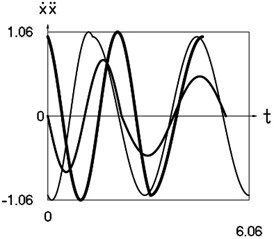d) Velocity multiplied by acceleration as function of time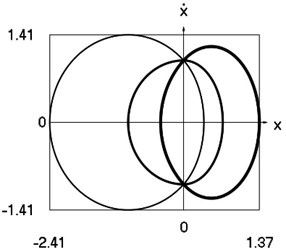e) Phase trajectory: velocity as function of displacement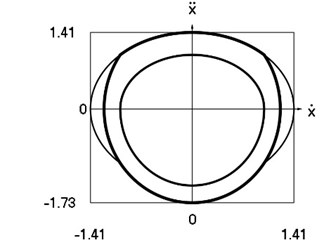f) Phase trajectory: acceleration as function of velocity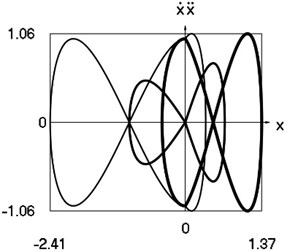g) Phase trajectory: velocity multiplied by acceleration as function of displacement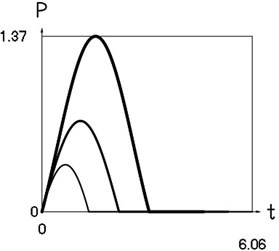h) Force as function of time

Simulation results for the investigated system are presented in Fig. 5.

## 6. Conclusions

Essentially nonlinear vibro-impact systems may have a number of various regimes of motion in steady state regimes under harmonic excitation. That couses unstable operation of such types of systems. Small variations of parameters or excitation jumps from one type of regime to another type of regime may occur. The purpose of this paper is to avoid this disadvantage in the behaviour of such system. This is achieved by choosing the fastening (the difference in the positions of statical equilibrium of impacting surfaces) between impacting members of the system to be equal to zero. All this is shown by the obtained formulas and graphical relationships, which were obtained by analytical and numerical methods.

On the basis of the presented results the qualities of dynamic behavior of the investigated essentially nonlinear vibrating system are revealed. Analytical relationships describing the motion of the system have been determined and are presented in the paper. Free damped vibrations, vibrations of the conservative system, time histories of motion as well as phase trajectories of motion for typical values of system parameters are presented in detail.

That opens a potential for the applicability of such systems in a wide variety of engineering applications.